# Grade 4 Linear Equations Solve linear equations with

• Slides: 17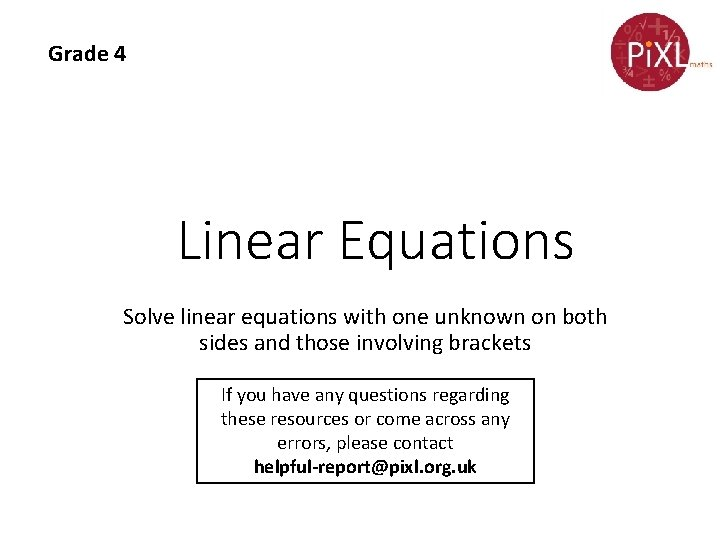Grade 4 Linear Equations Solve linear equations with one unknown on both sides and those involving brackets If you have any questions regarding these resources or come across any errors, please contact [email protected] org. uk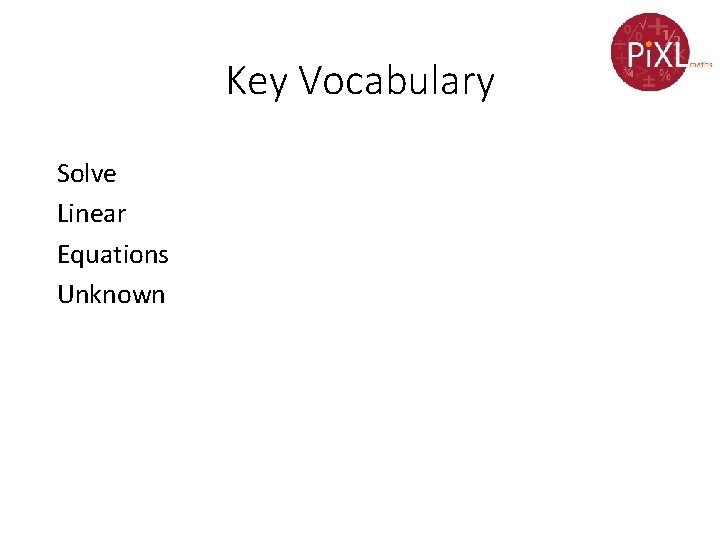Key Vocabulary Solve Linear Equations Unknown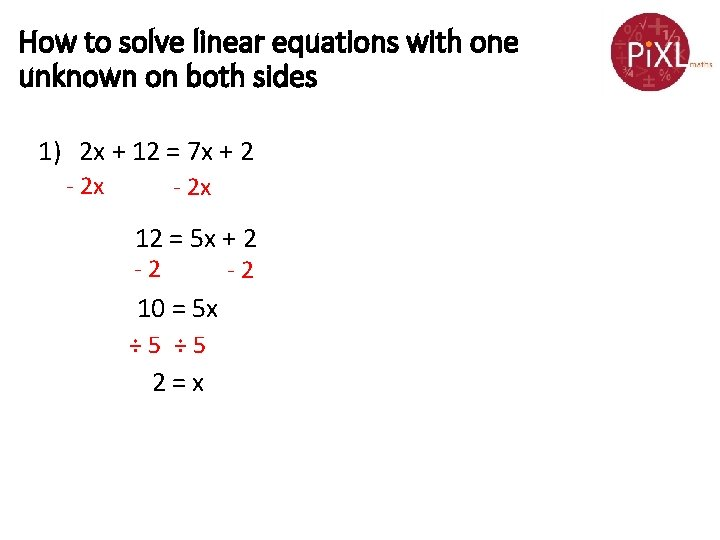How to solve linear equations with one unknown on both sides 1) 2 x + 12 = 7 x + 2 - 2 x 12 = 5 x + 2 -2 10 = 5 x ÷ 5 2=x -2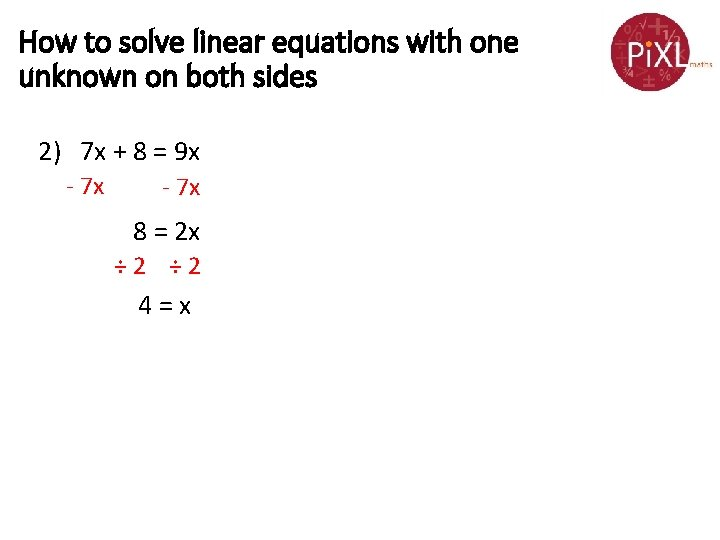How to solve linear equations with one unknown on both sides 2) 7 x + 8 = 9 x - 7 x 8 = 2 x ÷ 2 4=xHow to solve linear equations with one unknown on both sides involving brackets 3) 4 (y + 7) = 6 y 4 y + 28 = 6 y - 4 y 28 = 2 y ÷ 2 14 = y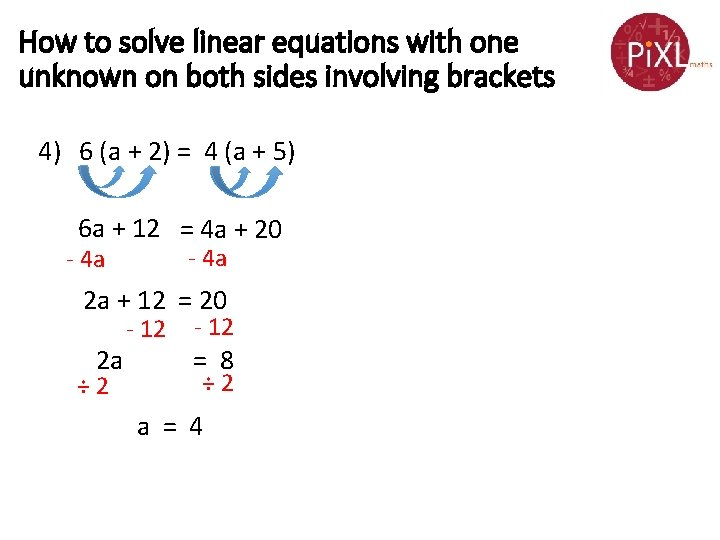How to solve linear equations with one unknown on both sides involving brackets 4) 6 (a + 2) = 4 (a + 5) 6 a + 12 = 4 a + 20 - 4 a 2 a + 12 = 20 2 a ÷ 2 - 12 = 8 ÷ 2 a = 4Solve linear equations – now you try. . . a) 2 x + 10 = 7 x + 5 b) 3 x + 9 = 6 x c) 3 (x + 4) = 6 x d) 8 y - 6 = 2(y + 9) e) 3(a + 4) = 5 (a + 2)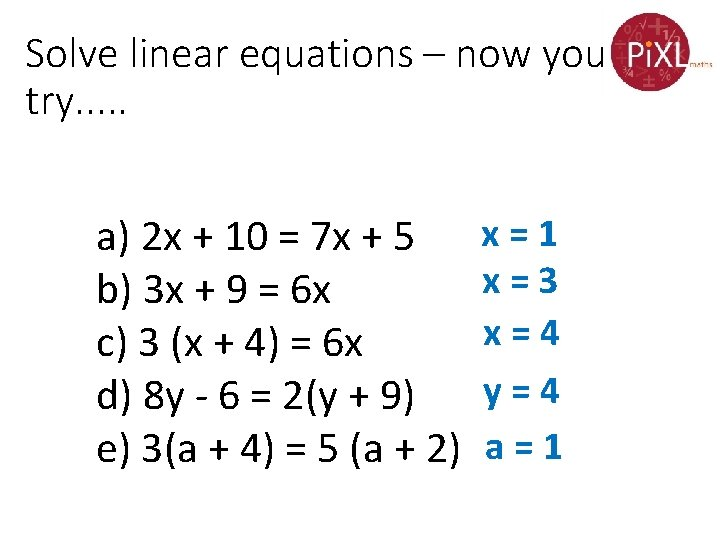Solve linear equations – now you try. . . a) 2 x + 10 = 7 x + 5 b) 3 x + 9 = 6 x c) 3 (x + 4) = 6 x d) 8 y - 6 = 2(y + 9) e) 3(a + 4) = 5 (a + 2) x=1 x=3 x=4 y=4 a=1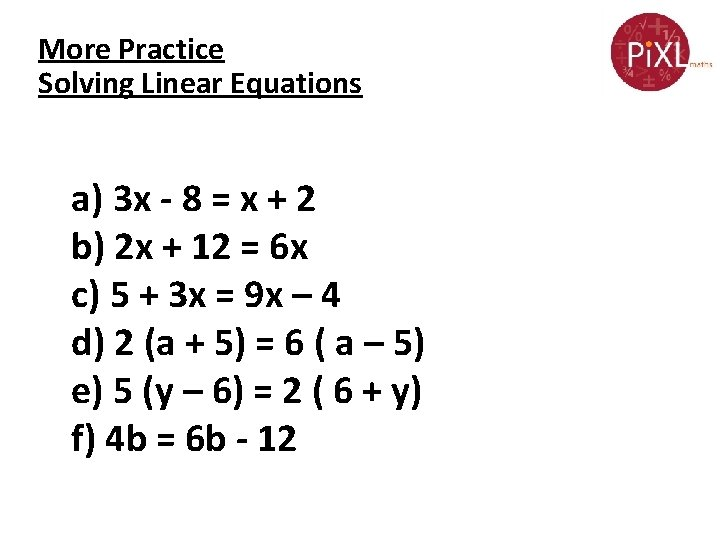More Practice Solving Linear Equations a) 3 x - 8 = x + 2 b) 2 x + 12 = 6 x c) 5 + 3 x = 9 x – 4 d) 2 (a + 5) = 6 ( a – 5) e) 5 (y – 6) = 2 ( 6 + y) f) 4 b = 6 b - 12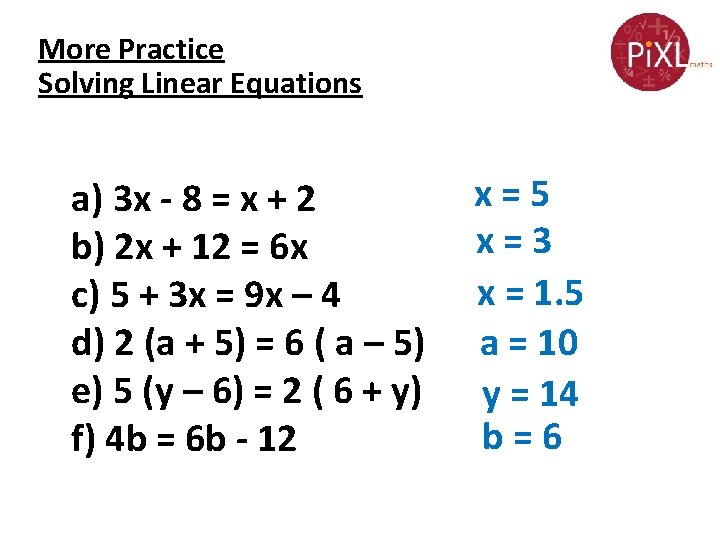More Practice Solving Linear Equations a) 3 x - 8 = x + 2 b) 2 x + 12 = 6 x c) 5 + 3 x = 9 x – 4 d) 2 (a + 5) = 6 ( a – 5) e) 5 (y – 6) = 2 ( 6 + y) f) 4 b = 6 b - 12 x=5 x=3 x = 1. 5 a = 10 y = 14 b=6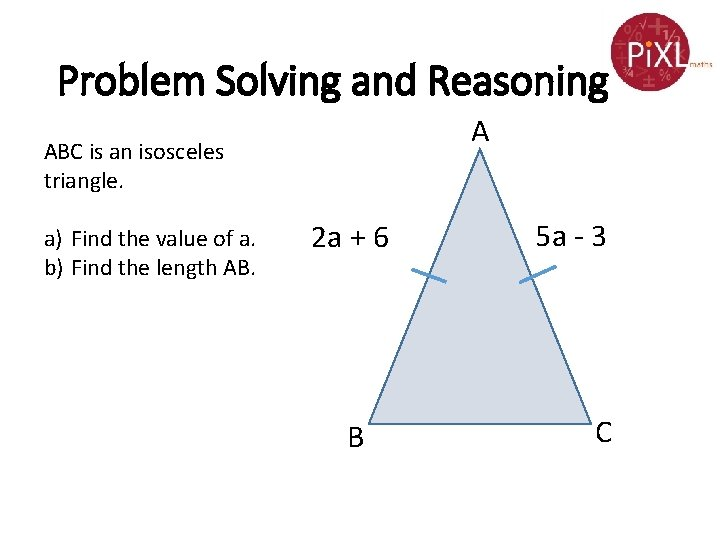Problem Solving and Reasoning A ABC is an isosceles triangle. a) Find the value of a. b) Find the length AB. 2 a + 6 B 5 a - 3 C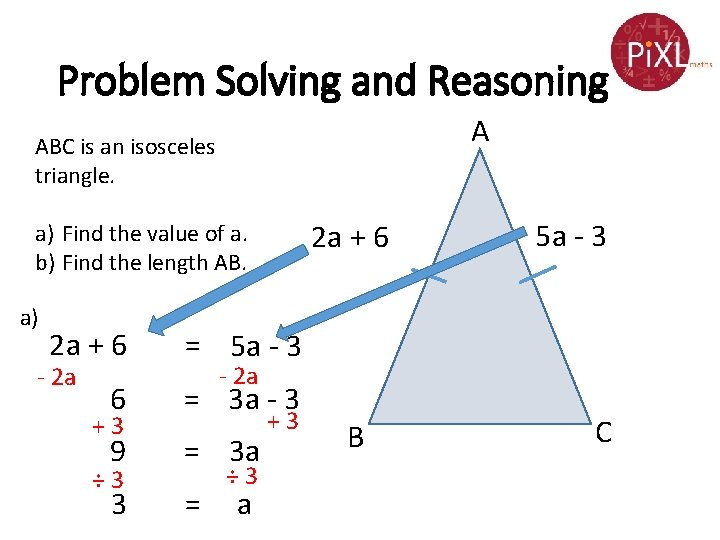Problem Solving and Reasoning A ABC is an isosceles triangle. 2 a + 6 a) Find the value of a. b) Find the length AB. a) 2 a + 6 = 5 a - 3 6 = 3 a - 3 9 = 3 a 3 = - 2 a +3 ÷ 3 5 a - 3 - 2 a ÷ 3 a +3 B C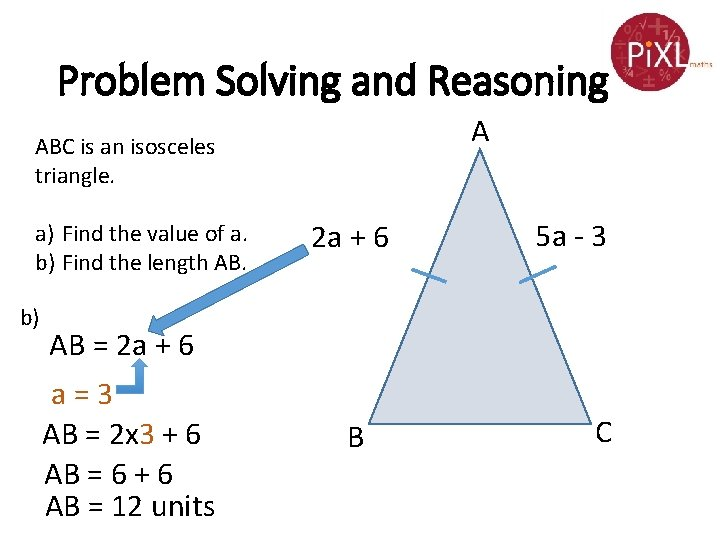Problem Solving and Reasoning A ABC is an isosceles triangle. a) Find the value of a. b) Find the length AB. b) 2 a + 6 5 a - 3 AB = 2 a + 6 a=3 AB = 2 x 3 + 6 AB = 6 + 6 AB = 12 units B C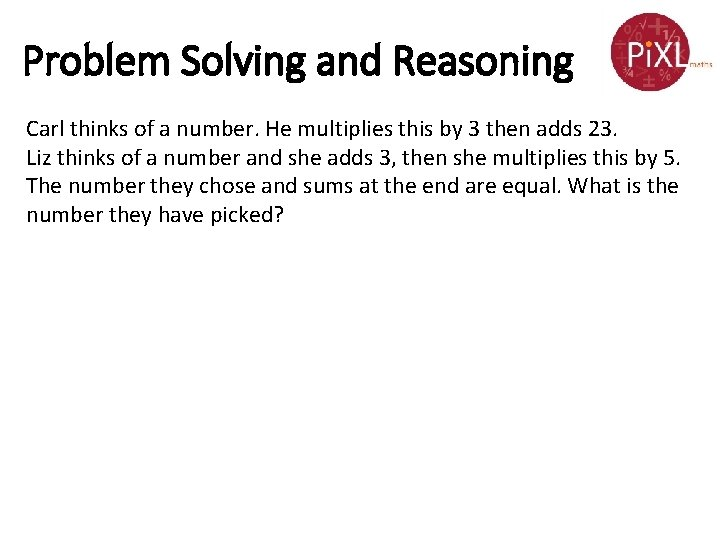Problem Solving and Reasoning Carl thinks of a number. He multiplies this by 3 then adds 23. Liz thinks of a number and she adds 3, then she multiplies this by 5. The number they chose and sums at the end are equal. What is the number they have picked?Problem Solving and Reasoning Carl thinks of a number. He multiplies this by 3 then adds 23. Liz thinks of a number and she adds 3, then she multiplies this by 5. The number they chose and sums at the end are equal. What is the number they have picked? They think of the same number to start with, lets say x. Carl’s expression => 3 x + 23 Liz’s expression => 5(x + 3) 3 x + 23 = 5 x + 15 23 = 2 x + 15 8 = 2 x 4=x They thought of number 4.Reason and explain • Explain why 3(2 x + 4) = 6(x + 1) cannot be solved. • Is there a way of checking whether our answers are correct? • Spot the mistake: 3 (b - 2) = 4 (b + 1) 3 b + 6 = 4 b + 4 - 3 b 6=b+4 -6 b= 2 -6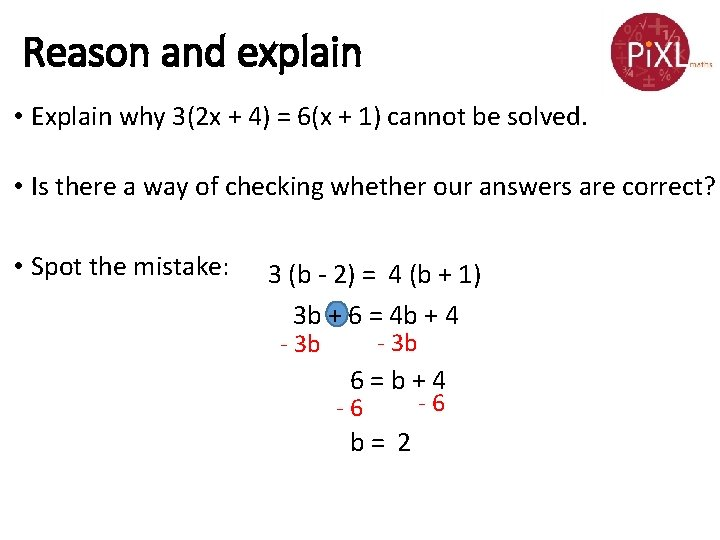Reason and explain • Explain why 3(2 x + 4) = 6(x + 1) cannot be solved. • Is there a way of checking whether our answers are correct? • Spot the mistake: 3 (b - 2) = 4 (b + 1) 3 b + 6 = 4 b + 4 - 3 b 6=b+4 -6 b= 2 -6